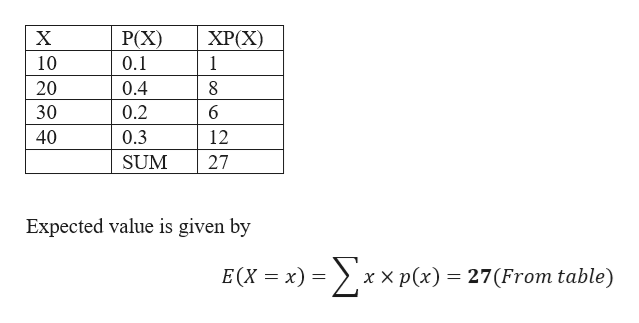# Given the following probability distribution:     X      P(X)     10    0.1     20    0.4     30    0.2     40    0.3What is the mean (expected value)?

Question
2 views
 Given the following probability distribution:      X      P(X)      10    0.1      20    0.4      30    0.2      40    0.3 What is the mean (expected value)?
check_circle

Step 1

Given data and calculation for the expec...help_outlineImage TranscriptioncloseP(X) XP(X) 10 0.1 1 0.4 20 0.2 30 40 0.3 12 27 SUM Expected value is given by E(X = x) = xx p(x) = 27(From table) fullscreen

### Want to see the full answer?

See Solution

#### Want to see this answer and more?

Solutions are written by subject experts who are available 24/7. Questions are typically answered within 1 hour.*

See Solution
*Response times may vary by subject and question.
Tagged in

### Other